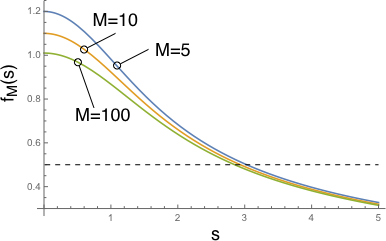# Discrete summation of Gaussian functions. Decay time problem

I am facing the following problem. I have a function which is defined through a discrete sum of Gaussians $$F_M(t) = 2\sum\limits_{n=1}^{M}e^{-t^2 \sigma^2 n^2}\times \sum\limits_{k=n}^{M}p_k p_{k-n} + \sum\limits_{k=0}^{M}p_k^2$$ where $\sigma$ is just a real parameter and $p_k$ are weights $$\sum\limits_{k=0}^{M}p_k = 1.$$ This functions decays from $F_M(0)=1$ down to $F_M(\infty)=\sum\limits_{k=0}^{M}p_k^2$.

The question is: how the decay time $\tau$ defined through the condition $F_M(\tau) = 1/2(1 - F_{M}(\infty))$ (at half height) depends on $M$?

Firstly, I tried to attack a particular case when $p_k = 1/(M+1)$. Then $$F_M(t) = \frac{2}{(M+1)^2}\left[(M+1)\sum\limits_{n=1}^{M}e^{-t^2 \sigma^2 n^2} - \sum\limits_{n=1}^{M}n\ e^{-t^2 \sigma^2 n^2} \right] + \frac{1}{(M+1)}.$$ I didn't manage to obtain any answer even for this simple case. The size $M$ does not necessarily has to be very large, but it is also interesting what happens when $M \rightarrow \infty$. Any ideas how the decay time might depend on $M$?

the decay time in your "simple case" is well approximated by the large-$$M$$ limit [*]

$$\lim_{M\rightarrow\infty}M\sigma\tau=2.84$$

here is a plot of

$$f_M(s)=\left.\frac{F_M(t)}{1-F_M(\infty)}\right|_{t=s/(M\sigma)}$$

for $$M=5,10,100$$, that shows the half-time $$s\approx 3$$ is quite accurate already for not so large values of $$M$$[*] for the large-$$M$$ limit I replace the sum in the defition of $$F_M(t)$$ by an integral and solve for the half-time,

$$\int_0^M\frac{2(M-x)}{M^2}e^{-(\sigma \tau x)^2}\,dx=\frac{1}{2}$$

this gives $$M\sigma\tau=2.84092\cdots$$.